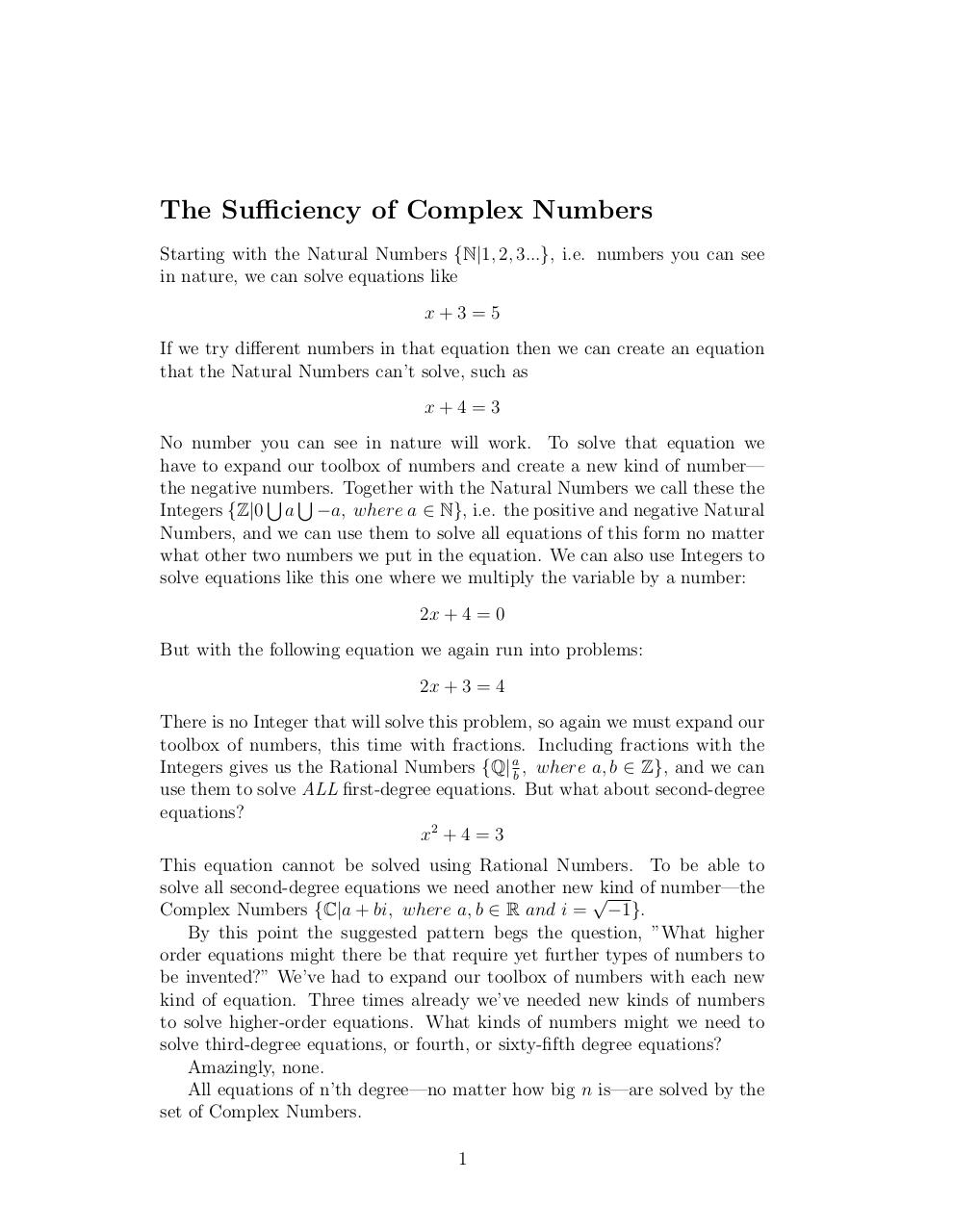# complex .pdf

### File information

Original filename: complex.pdf

This PDF 1.5 document has been generated by TeX / pdfTeX-1.40.18, and has been sent on pdf-archive.com on 03/05/2020 at 04:59, from IP address 24.8.x.x. The current document download page has been viewed 125 times.
File size: 82 KB (1 page).
Privacy: public file

complex.pdf (PDF, 82 KB)

### Document preview

The Sufficiency of Complex Numbers
Starting with the Natural Numbers {N|1, 2, 3...}, i.e. numbers you can see
in nature, we can solve equations like
x+3=5
If we try different numbers in that equation then we can create an equation
that the Natural Numbers can’t solve, such as
x+4=3
No number you can see in nature will work. To solve that equation we
have to expand our toolbox of numbers and create a new kind of number—
the negative numbers.
Together with the Natural Numbers we call these the
S S
Integers {Z|0 a −a, where a ∈ N}, i.e. the positive and negative Natural
Numbers, and we can use them to solve all equations of this form no matter
what other two numbers we put in the equation. We can also use Integers to
solve equations like this one where we multiply the variable by a number:
2x + 4 = 0
But with the following equation we again run into problems:
2x + 3 = 4
There is no Integer that will solve this problem, so again we must expand our
toolbox of numbers, this time with fractions. Including fractions with the
Integers gives us the Rational Numbers {Q| ab , where a, b ∈ Z}, and we can
use them to solve ALL first-degree equations. But what about second-degree
equations?
x2 + 4 = 3
This equation cannot be solved using Rational Numbers. To be able to
solve all second-degree equations we need another new√kind of number—the
Complex Numbers {C|a + bi, where a, b ∈ R and i = −1}.
By this point the suggested pattern begs the question, ”What higher
order equations might there be that require yet further types of numbers to
be invented?” We’ve had to expand our toolbox of numbers with each new
kind of equation. Three times already we’ve needed new kinds of numbers
to solve higher-order equations. What kinds of numbers might we need to
solve third-degree equations, or fourth, or sixty-fifth degree equations?
Amazingly, none.
All equations of n’th degree—no matter how big n is—are solved by the
set of Complex Numbers.
1#### HTML Code

Copy the following HTML code to share your document on a Website or Blog

#### QR Code### Related keywords